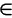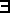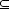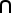# Maths - Set Related Proofs

On these pages we look at proofs about sets. In mathematics 'set' is a fundamental concept but thare are different approaches that can be taken to it:

• In 'set theory' everything is a set.
• Often we may look at set as a sort of minimal structure onto which we can add extra structure.
• In computing a set may be seen as a collection, like a list, where there are no duplicates and order is not important.

There is a correspondance between set theory and second order logic (and therefore HOL) although, on this page, we are looking at logic to prove things about sets rather than investigating this correspondance. There is a lot of built in support in Isabelle for working with sets and the notation of sets.

Here we will treat set as a mimimal structure, with the concept of set membership and subsets, onto which we can add other structures.

This page is a part of the site (starting on this page) where we are using a program called Isabelle to construct proofs on various structures.

In Isabelle sets are typed. All elements have the same type (say τ) and the set itself has the type τ set (the order of type constructors is reversed compared to many languages). A type variable a is written: 'a so a set of these is written: 'a set.

## Concepts

### Finite Set Notation

Isabelle allows finite sets to be written like:

{a,b,c}

examples

Isabelle Notation Meaning
a ∈ A A is a member of B
a ∉ A A is not a member of B
{} empty set
UNIV universal set
{x. P}

comprehension

set of all elements that satisfy predicate P

{p*q | p q. pprime /\ qprime}

more complicated comprehension in this case all numbers which are the products of two primes. Could also be represented by:

{x.p q. z = p*q /\ pprime /\ qprime}

AB A is a subset of B

### Set Membership

the Isabelle keyword axiomatization is a form of typedef which has axioms.

• Collect infers the members of a set from a predicate.
• member takes a element and a set and returns true if the element is a member of the set.
 ```axiomatization Collect :: "('a => bool) => 'a set" -- "comprehension" and member :: "'a => 'a set => bool" -- "membership" where mem_Collect_eq [iff, code_unfold]: "member a (Collect P) = P a" and Collect_mem_eq [simp]: "Collect (λx. member x A) = A" notation (HTML output) member ("op ∈") and member ("(_/ ∈ _)" [51, 51] 50) and not_member ("op ∉") and not_member ("(_/ ∉ _)" [51, 51] 50)```

The axioms show Collect and member as having inverse functions. that is, Collect allows us to compose a set and member allows us to decompose it.

In category theory a function a subobject classifier has the type: 'a set => bool.

Set comprehension is a construction that allows us to define a set by stating the properties of its members.

### Subset

AB means A is a subset of B

AB iffxA : xB

Isabelle code:

 ```lemma subsetD [elim, intro?]: "A ⊆ B ==> c ∈ A ==> c ∈ B" by (simp add: less_eq_set_def le_fun_def) -- {* Rule in Modus Ponens style. *} lemma rev_subsetD [no_atp,intro?]: "c ∈ A ==> A ⊆ B ==> c ∈ B" -- {* The same, with reversed premises for use with @{text erule} -- cf @{text rev_mp}. *} by (rule subsetD)```

#### Transitivity of Subset

AB and BC then AC

 ```lemma psubset_subset_trans: "A ⊂ B ==> B ⊆ C ==> A ⊂ C" by (auto simp add: psubset_eq)```

#### Equivilance

if P implies Q and Q implies P then P is equivalent to Q written: P iff Q

 ```lemma set_eqI: assumes "!!x. x ∈ A <-> x ∈ B" shows "A = B" proof - from assms have "{x. x ∈ A} = {x. x ∈ B}" by simp then show ?thesis by simp qed lemma set_eq_iff [no_atp]: "A = B <-> (∀x. x ∈ A <-> x ∈ B)" by (auto intro:set_eqI) lemma set_eq_subset: "(A = B) = (A ⊆ B & B ⊆ A)" by blast lemma subset_iff [no_atp]: "(A ⊆ B) = (∀t. t ∈ A --> t ∈ B)" by blast lemma subset_iff_psubset_eq: "(A ⊆ B) = ((A ⊂ B) | (A = B))" by (unfold less_le) blast ```

#### Inference Rule

Proving forall statement:

let a be an arbitary chosen element of A

to proveaA:P(a)

we need to prove P(a) for a

 ```lemma ballI [intro!]: "(!!x. x:A ==> P x) ==> ALL x:A. P x" by (simp add: Ball_def)```

#### Negation

¬aA:P(a) is equivalent toaA:¬P(a)

¬aA:P(a) is equivalent toaA:¬P(a)

#### Union and Intersection

if AC and BC then ABC

if AC and BC then A U BC

 ```text {* \medskip Finite Union -- the least upper bound of two sets. *} lemma Un_upper1: "A ⊆ A ∪ B" by (fact sup_ge1) lemma Un_upper2: "B ⊆ A ∪ B" by (fact sup_ge2) lemma Un_least: "A ⊆ C ==> B ⊆ C ==> A ∪ B ⊆ C" by (fact sup_least) text {* \medskip Finite Intersection -- the greatest lower bound of two sets. *} lemma Int_lower1: "A ∩ B ⊆ A" by (fact inf_le1) lemma Int_lower2: "A ∩ B ⊆ B" by (fact inf_le2) lemma Int_greatest: "C ⊆ A ==> C ⊆ B ==> C ⊆ A ∩ B" by (fact inf_greatest)```

Axioms

 ```text {* \medskip @{text Un}. *} lemma Un_absorb: "A ∪ A = A" by (fact sup_idem) (* already simp *) lemma Un_left_absorb: "A ∪ (A ∪ B) = A ∪ B" by (fact sup_left_idem) lemma Un_commute: "A ∪ B = B ∪ A" by (fact sup_commute) lemma Un_left_commute: "A ∪ (B ∪ C) = B ∪ (A ∪ C)" by (fact sup_left_commute) lemma Un_assoc: "(A ∪ B) ∪ C = A ∪ (B ∪ C)" by (fact sup_assoc) lemmas Un_ac = Un_assoc Un_left_absorb Un_commute Un_left_commute -- {* Union is an AC-operator *} lemma Un_absorb1: "A ⊆ B ==> A ∪ B = B" by (fact sup_absorb2) lemma Un_absorb2: "B ⊆ A ==> A ∪ B = A" by (fact sup_absorb1) lemma Un_empty_left: "{} ∪ B = B" by (fact sup_bot_left) (* already simp *) lemma Un_empty_right: "A ∪ {} = A" by (fact sup_bot_right) (* already simp *) lemma Un_UNIV_left: "UNIV ∪ B = UNIV" by (fact sup_top_left) (* already simp *) lemma Un_UNIV_right: "A ∪ UNIV = UNIV" by (fact sup_top_right) (* already simp *) lemma Un_insert_left [simp]: "(insert a B) ∪ C = insert a (B ∪ C)" by blast lemma Un_insert_right [simp]: "A ∪ (insert a B) = insert a (A ∪ B)" by blast lemma Int_insert_left: "(insert a B) Int C = (if a ∈ C then insert a (B ∩ C) else B ∩ C)" by auto lemma Int_insert_left_if0[simp]: "a ∉ C ==> (insert a B) Int C = B ∩ C" by auto lemma Int_insert_left_if1[simp]: "a ∈ C ==> (insert a B) Int C = insert a (B Int C)" by auto lemma Int_insert_right: "A ∩ (insert a B) = (if a ∈ A then insert a (A ∩ B) else A ∩ B)" by auto lemma Int_insert_right_if0[simp]: "a ∉ A ==> A Int (insert a B) = A Int B" by auto lemma Int_insert_right_if1[simp]: "a ∈ A ==> A Int (insert a B) = insert a (A Int B)" by auto lemma Un_Int_distrib: "A ∪ (B ∩ C) = (A ∪ B) ∩ (A ∪ C)" by (fact sup_inf_distrib1) lemma Un_Int_distrib2: "(B ∩ C) ∪ A = (B ∪ A) ∩ (C ∪ A)" by (fact sup_inf_distrib2) lemma Un_Int_crazy: "(A ∩ B) ∪ (B ∩ C) ∪ (C ∩ A) = (A ∪ B) ∩ (B ∪ C) ∩ (C ∪ A)" by blast lemma subset_Un_eq: "(A ⊆ B) = (A ∪ B = B)" by (fact le_iff_sup) lemma Un_empty [iff]: "(A ∪ B = {}) = (A = {} & B = {})" by (fact sup_eq_bot_iff) (* FIXME: already simp *) lemma Un_subset_iff [no_atp, simp]: "(A ∪ B ⊆ C) = (A ⊆ C & B ⊆ C)" by (fact le_sup_iff) lemma Un_Diff_Int: "(A - B) ∪ (A ∩ B) = A" by blast lemma Diff_Int2: "A ∩ C - B ∩ C = A ∩ C - B" by blast ```

#### Introduction Rules

and or implication
 A B A/\B
(/\I)
 A A\/B
(\/I1)
 B A\/B
(\/I2)
 [A] ... B A=>B
(=>I)
 ```text {* \medskip Classical introduction rule: no commitment to @{prop A} vs @{prop B}. *} lemma UnCI [intro!]: "(c~:B ==> c:A) ==> c : A Un B" by auto lemma insert_def: "insert a B = {x. x = a} ∪ B" by (simp add: insert_compr Un_def) lemma mono_Un: "mono f ==> f A ∪ f B ⊆ f (A ∪ B)" by (fact mono_sup)```

#### Elimination Rules

and or implication
 A/\B A
(/\E1)
 A/\B B
(/\E2)
 [A] ... [B] ... A\/B C C
C
(\/E)
 A A=>B B
(=>E)
 ```lemma UnE [elim!]: "c : A Un B ==> (c:A ==> P) ==> (c:B ==> P) ==> P" by (unfold Un_def) blast lemma subsetCE [no_atp,elim]: "A ⊆ B ==> (c ∉ A ==> P) ==> (c ∈ B ==> P) ==> P" -- {* Classical elimination rule. *} by (auto simp add: less_eq_set_def le_fun_def)```